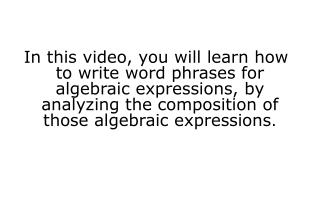Download Presentationv ariable – letter that can represent one or more numbers

# v ariable – letter that can represent one or more numbers - PowerPoint PPT PresentationDownload Presentation## v ariable – letter that can represent one or more numbers

- - - - - - - - - - - - - - - - - - - - - - - - - - - E N D - - - - - - - - - - - - - - - - - - - - - - - - - - -
##### Presentation Transcript

1. In this video, you will learn how to write word phrases for algebraic expressions, by analyzing the composition of those algebraic expressions.

2. algebraic expression – a mathematical phrase that contains a combination of numbers, variables, and operational symbols (+, , x, ) Variable – symbols (letters) than can represent one or more numbers.

3. variable – letter that can represent one or more numbers

4. Words to represent addition: increased by plus more than sum added to

5. Translating an expression with addition: d + 2 The sum of d and 2 2 more than d d increased by 2

6. Translating an expression with addition: d + 2 The sum of d dollars and 2 2 more than d drums d degrees increased by 2

7. Translating an expression with addition: d + 2 The sum of d dollars and 2 2 more than d drums d degrees increased by 2

8. Translating an expression with addition: d + 2 The sum of d dollars and 2 2 more than d drums d degrees increased by 2

9. Words to represent subtraction: fewer than less than difference between decreased by ORDER MATTERS!

10. Words to represent subtraction: fewer than less than difference between decreased by ORDER MATTERS!

11. Some examples involving subtraction: h – 5 the difference between h hens and 5 5 less than h hippos h hearts decreased by 5

12. Some examples involving subtraction: h – 5 the difference between h hens and 5 5 less than h hippos h hearts decreased by 5

13. Some examples involving subtraction: h – 5 the difference between h hens and 5 5 less than h hippos h hearts decreased by 5

14. Some examples involving subtraction: h – 5 the difference between h hens and 5 5 less than h hippos h hearts decreased by 5

15. Words that suggest multiplication: the product of times multiplied by ORDER MATTERS!

16. Some examples involving multiplication: 8f 8 times the f feet the product of 8 and f frogs 8 multiplied by ffingers

17. Some examples involving multiplication: 8f 8 times the f feet the product of 8 and f frogs 8 multiplied by ffingers

18. Some examples involving multiplication: 8f 8 times the f feet the product of 8 and f frogs 8 multiplied by ffingers

19. Some examples involving multiplication: 8f 8 times the f feet the product of 8 and f frogs 8 multiplied by ffingers

20. Words that suggest division: quotient of divided by divided among shared between O ORDS!

21. Words that suggest division: quotient of divided by divided among shared between ORDER MATTERS!

22. Some examples involving division: d 3 d dollars divided amongst 3 friends d donuts shared between 3 people the quotient of d dear and 3

23. A review of clue words:

24. Some examples involving all operations: 12 more than g glasses

25. In this video, you learned how to write word phrases for algebraic expressions, by analyzing the composition of those algebraic expressions.